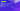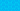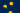Pricing# Pretax Profit Margins Explained

Written by

The pretax profit margin reflects the level of profit a company generates before it pays its taxes. It is calculated from the information given on a company’s income statement.

## Understanding profit margins

Profit margins are also known as profitability ratios. They are a measure of how well a business converts revenue into profit. The most common profit margins are the gross profit margin, the operating profit margin, the pretax profit margin, and the net profit margin.

Gross profit margins tend to be most useful to managers analyzing their product/service offering. Investors generally focus on the pretax profit margin and the net profit margin. They may also look at the operating profit margin.

## What does pretax mean?

The phrase “pretax” refers to the standard layout of company income statements. These have to follow a consistent model of presentation starting with revenue and ending with net income:

• Revenue

• Cost of Goods Sold/Cost of Sales

• Operating Expenses

• Depreciation and Amortization

• Interest (income and expenses)

• Other Income and Expenses

• Tax

• Net Income

Tax is always the last expense to be deducted. This means that pretax is essentially shorthand for “all income and expenses other than tax.”

## The pretax profit margin formula

The pretax profit margin is calculated by the formula:

Income Before Taxes divided by Revenue multiplied by 100

In other words, you take the gross revenue, subtract all expenses down to Other Expenses (inclusive) and, if relevant, add on interest income. You divide this figure by the gross revenue (i.e. the top line) and then multiply the result by 100.

## The net profit margin formula

The net profit margin is calculated by the formula:

Net Income divided by Revenue multiplied by 100

In other words, you divide the bottom line by the top line and multiply the result by 100.

## Pretax profit margin versus net profit margin

The pretax profit margin essentially reflects how much profit a company generates before the government takes its share. The net profit margin reflects how much profit is left for shareholders after the government has taken its share. Both are useful to know but they serve different purposes.

Pretax profit margins largely reflect the impact of management decisions. There is some nuance here – for example, poor weather can lead to a poor harvest and hence increase a company’s pretax expenses. These types of issues will, however, typically impact all companies in the same industry in much the same way.

Net profit margins, by contrast, reflect where a company stands after it has paid everything it owes to the government. For the most part, companies have very little room to maneuver here. On the one hand, this means that tax expenses cannot usually be taken as a measure of the management team’s abilities. On the other hand, investors do need to take it into consideration.

## Pretax profit margin versus operating profit margin

The operating profit margin is calculated using the formula:

Operating Income divided by Revenue multiplied by 100

Operating Income is calculated by subtracting Cost of Goods Sold/Cost of Sales and Operating Expenses from Revenue.

The operating profit margin can be useful for comparing businesses that operate in the same sector but have different business models. For example, say Company A and Company B are direct competitors.

Company A chooses to buy its fixed assets but Company B chooses to lease them. This means that Company A has depreciation costs whereas Company B does not. In this situation, the operating profit margin could prove a more useful point of comparison than either the pretax profit margin or the net profit margin.

## How we can help

If you’re interested in finding out more about pretax profit margins, then get in touch with our financial experts. Find out how GoCardless can help you with ad hoc payments or recurring payments.

Interested in automating the way you get paid? GoCardless can help

Contact salesSales

Contact sales

+1(415) 523-2279

Support

help@gocardless.com